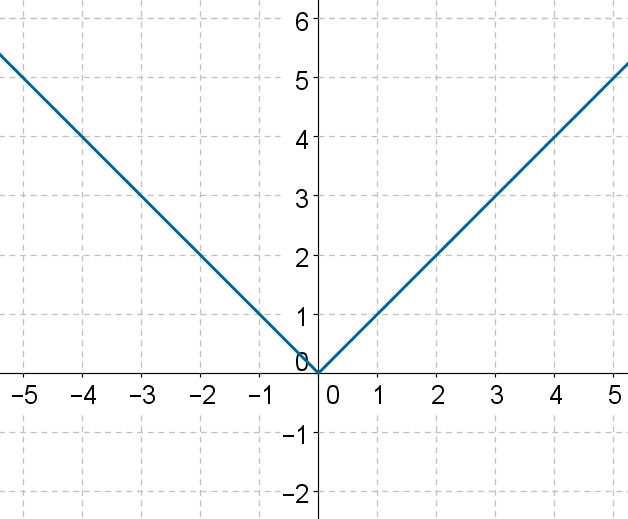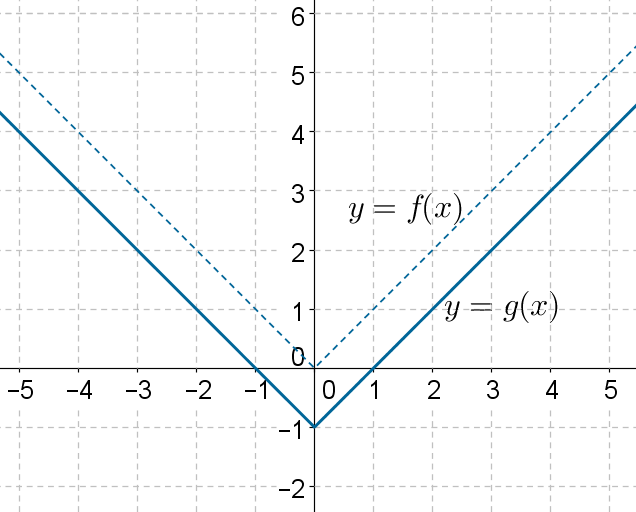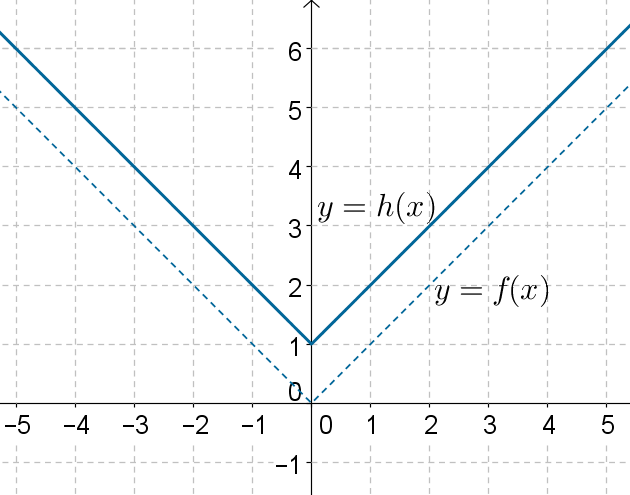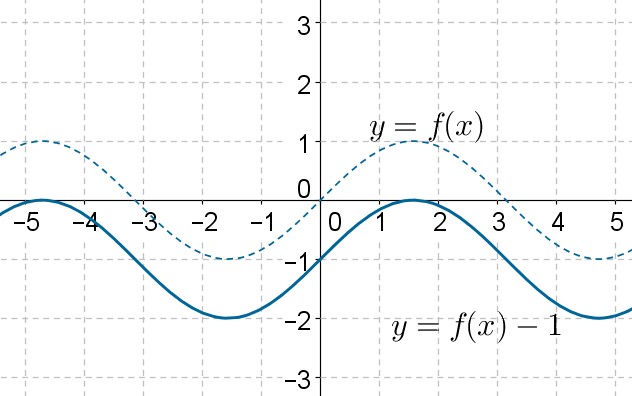# Vertical Translation

Go back to  'Functions'

The following is the graph of $$f\left( x \right) = \left| x \right|$$, as we have seen earlier:Using this graph, can we plot the graph of $$g\left( x \right) = \left| x \right|- 1$$?

Think carefully: what will be the relation between the graphs of f and g? For any value of the input x, the output produced by g will be 1 less than the output produced by f, because.

$g\left( x \right) = \left| x \right| - 1 = f\left(x \right) - 1$

This means that we can obtain the graph of g by simply down-shifting the graph of f by 1 unit:Similarly, the graph of $$h\left( x \right) = \left| x \right| + 1 = f\left( x\right) + 1$$ can be obtained by up-shifting the graph of f by 1 unit:In general, the graph of $$y = f\left( x \right) + k$$ can be obtained by vertically shifting the graph of $$y = f\left( x\right)$$ by $$\left| k \right|$$units . The direction of the shift will depend upon the sign of k. If is positive, the shift will be upward, else it will be downward.

The following figure shows the graph of a function $$y = f\left( x \right)$$       (dotted)and the graph of $$y = f\left( x \right) - 1$$ obtained by down-shifting the graph of $$y =f\left( x \right)$$ by 1 unit:Functions
Functions
grade 10 | Questions Set 2
Functions
Functions
grade 10 | Questions Set 1